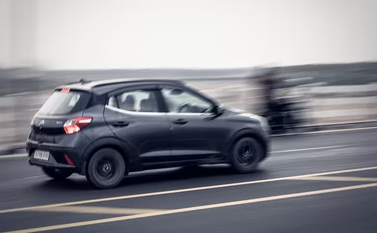## Constant Acceleration

A car accelerates at a constant rate of 12 m/sec^2. The car travels 140 meters while its speed changes to 60 m/sec. What is the initial velocity?Hint
$$v^{2}=v_{o}^{2}+2a(S-S_{o})$$$where $$v$$ is the velocity along the direction of travel, $$v_o$$ is the velocity at time $$t_0$$ , $$a$$ is constant acceleration, $$S$$ is the displacement at time $$t$$ along the line of travel, and $$S_o$$ is the displacement at time $$t_0$$ . Hint 2 Solve for initial velocity, $$v_o$$ . For constant acceleration, the equation for velocity as a function of position: $$v^{2}=v_{o}^{2}+2a(S-S_{o})$$$
where $$v$$ is the velocity along the direction of travel, $$v_o$$ is the velocity at time $$t_0$$ , $$a$$ is constant acceleration, $$S$$ is the displacement at time $$t$$ along the line of travel, and $$S_o$$ is the displacement at time $$t_0$$ .
Solving for initial velocity:
$$v_{o}^{2}=v^{2}-2a(S-S_{o})$$$$$v_{o}^{2}=(60\:m/sec)^{2}-2(12\:m/sec^2)(140\:m)$$$
$$v_{o}^{2}=3,600\:m^2/sec^{2}-3,360\:m^2/sec^2$$$$$v_{o}=\sqrt{240\:m^2/sec^2}= 15.5\:m/sec$$$
15.5 m/s